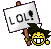# question 11 n9 trial##question 11 n9 trial

why the volume of oxygen is 12dm3???
why not 24 dm3??##hm.suai

thats why .i also dont know how to do this question .##Re: question 11 n9 trial

because oxygen has 1 mole.hydrogen peroxide has 2 mole. so must divide by two

##Re: question 11 n9 trial

i answered 48 dm3..... who got the same answer with me?##Re: question 11 n9 trial

OH OK! like that ahhh,##Guide to answer Question 11

Look at the chemical equation.

1) What is the relationship between number of moles hydrogen peroxide & oxygen gas?
2) When one mole of hydrogen peroxide is decomposed, find the moles of oxygen gas produced.
3) Find the volume of gas by using relationship with Avogadro Constant

##Question 11

2 mol of H2O2 produce
1 mol of O2
so volume of 1 mol of gas is 24 dm3
then volume of 1 mol of O2 is
24 divide 2 = 12dm3

##hmm.....

i got it correct...
check no of mole die btol2
2 mol of H2O2 = 1 mol of O
hence.. 1 mol of H2O2 = 1/2 mol of O .. okayhazie noo
Guest

##Re: question 11 n9 trial

my answer is 24..
but now i understandthanks !

##Re: question 11 n9 trial

got the answer already..tq..##Re: question 11 n9 trial

0.5x24= 12.. you have to do based on the equation... then..u can see the works clearly..##Re: question 11 n9 trial

Sponsored contentPermissions in this forum:
You cannot reply to topics in this forum